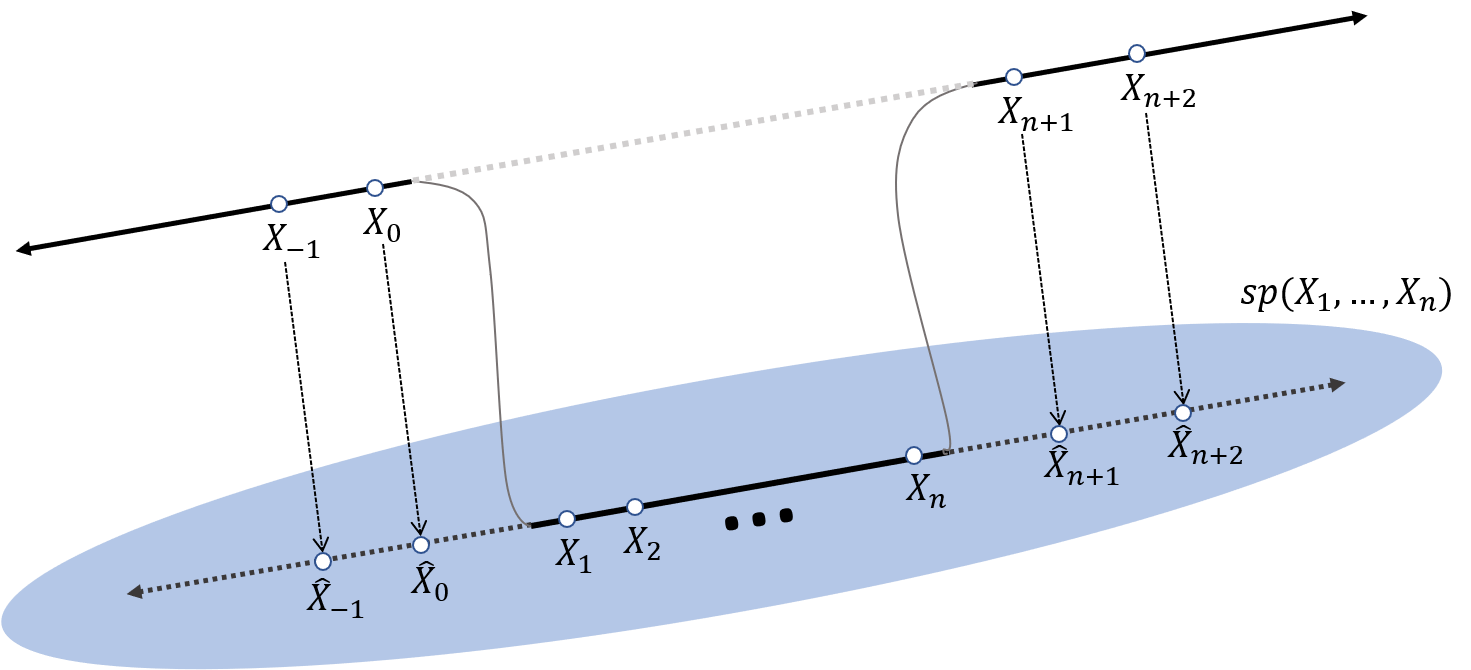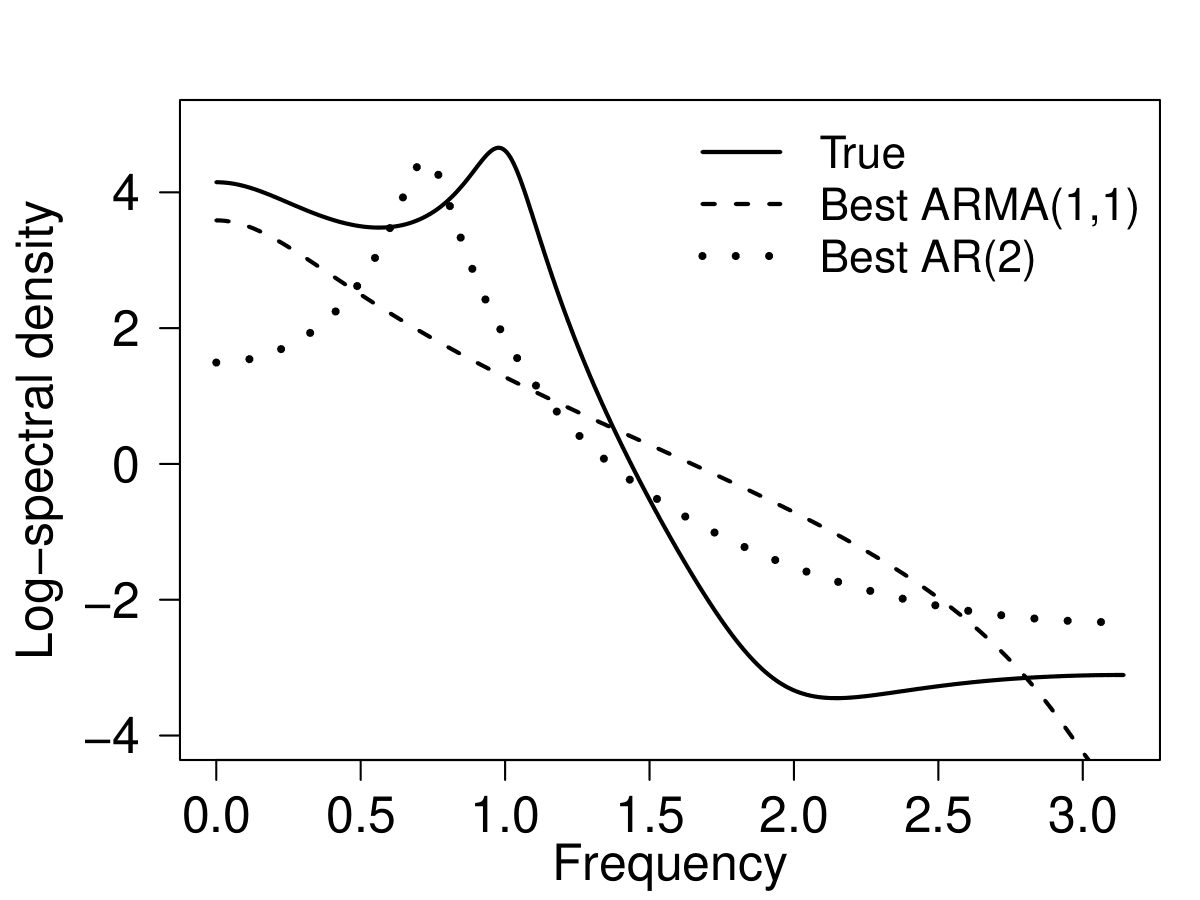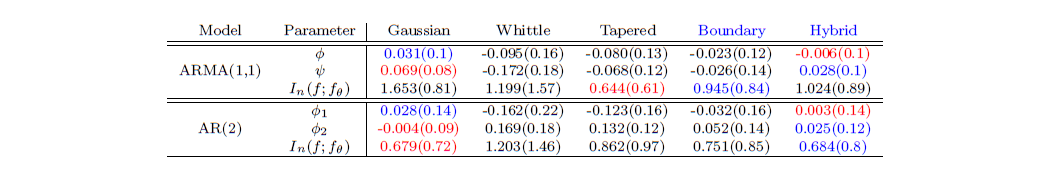Want to share your content on R-bloggers? click here if you have a blog, or here if you don't.

## Overview

Suppose $$\{X_t: t\in \mathbb{Z}\}$$ is a second order stationary time series where $$c(r) = \text{cov}(X_{t+r},X_t)$$ and $$f(\omega) = \sum_{r\in\mathbb{Z}}c(r)e^{ir\omega}$$ are the corresponding autocovariance and spectral density function, respectively. For notational convenience, we assume the time series is centered, that is $$\textrm{E}(X_t)=0$$. Our aim is to fit a parametric second-order stationary model (specified by $$\{c_{f_\theta}(r)\}$$ or $$f_\theta(\omega)$$) to the observed time series $$\underline{X}_n = (X_1, …, X_n)^\top$$. There are two classical estimation methods based on the quasi-likelihood criteria. The first is a time-domain method which minimizes the (negative log) quasi Gaussian likelihood $\begin{equation} \mathcal{L}_n(\theta) = \frac{1}{n}\big(\underline{X}_n^{\top} \Gamma_n(f_\theta)\underline{X}_n + \log \det\Gamma_n(f_\theta) \big), \end{equation}$ where $$[\Gamma_n(f_\theta)]_{s,t} = c_{f_\theta}(s-t)$$ is a covariance matrix of $$\underline{X}_n$$. The second is a frequency-domain method which minimizes the Whittle likelihood $\begin{equation} K_n(\theta) = \frac{1}{n}\sum_{k=1}^{n}\bigg(\frac{|J_n(\omega_k)|^2}{f_\theta(\omega_k)} + \log f_\theta(\omega_k)\bigg) \qquad \omega_k = \frac{2\pi k}{n}, \end{equation}$ where $$J_n(\omega_k) =n^{-1/2}\sum_{t=1}^{n} X_t e^{it\omega_k}$$, $$1 \leq k \leq n$$, is the discrete Fourier transform (DFT) of the (observed) time series.

The Whittle likelihood is computationally a very attractive method. Thus, it has become a popular method for parameter estimation of both long and short memory stationary time series. Moreover, the Whittle likelihood has gained traction as a quasi-likelihood between the periodogram and conjectured spectral density.

Despite its advantages, it is well-known that for small samples, the Whittle likelihood can give rise to estimators with a substantial bias. Dahlhaus (1988) showed that the finite sample bias in the periodogram impacts the performance of the Whittle likelihood. To remedy this, he proposed the tapered Whittle likelihood based on the tapered periodogram. He proved that the tapered periodogram led to a significant reduction in bias.

However, as far as we are aware, there are no results that explain the “precise difference” between the Gaussian likelihood and the Whittle likelihood. The objective of our paper to bridge the gap between the time- and frequency-domain approaches by deriving an exact expression of the difference between the Gaussian and Whittle likelihood.

The Contribution of this paper is three-fold:

• We obtain a linear transformation (which we named the complete DFT) that is “biorthogonal” to the DFT of an observed time series.

• We use the complete DFT to rewrite the Gaussian likelihood in the frequency domain.

• Using an approximation for the difference, we propose a new frequency domain quasi-likelihood criteria — the boundary corrected Whittle and the hybrid Whittle likelihood.

In the following sections, we summarize each contribution.

## The complete DFT

We introduce our main theorem which obtains a transform that is biorthogonal to the DFT.

Theorem 1 (The biorthogonal transform) Let $$\{X_t\}$$ be a centered second order stationary time series with spectral density $$f$$ which is bounded and strictly positive. For $$\tau \in \mathbb{Z}$$, let $$\hat{X}_{\tau,n}$$ denote the best linear predictors of $$X_\tau$$ given $$\underline{X}_n$$. Let $$\widetilde{J_n}(\omega;f) = J_n(\omega) + \widehat{J_n}(\omega;f)$$ be the complete DFT where $\begin{equation*} \widehat{J_n}(\omega;f) = n^{-1/2} (\sum_{\tau \leq 0} \hat{X}_{\tau,n}e^{i\tau \omega} + \sum_{\tau > n} \hat{X}_{\tau,n}e^{i\tau \omega} ). \end{equation*}$ Then, $\begin{equation*} \text{cov}(\widetilde{J_n}(\omega_{k_1};f), J_n(\omega_{k_2})) = f(\omega_{k_1}) \delta_{k_1 = k_2} \quad 1\leq k_1, k_2 \leq n, \end{equation*}$ where $$\delta_{k_1 = k_2} = 1$$ if $$k_1=k_2$$ and zero otherwise.

From the above theorem, the biorthogonal transform corresponding to the regular DFT—henceforth called the complete DFT—contains the regular DFT plus the Fourier transform of the best linear predictors outside the domain of observation. A visualization of the observations and the predictors that are involved in the construction of the complete DFT is given below.## Contrasting the Gaussian and Whittle likelihood

Using the complete DFT, we can represent the Gaussian likelihood in the frequency domain.

Theorem 2 (A frequency domain representation of the Gaussian likelihood) The quadratic term in the Gaussian likelihood can be rewritten as $\begin{equation*} \mathcal{L}_n(\theta) = \frac{1}{n}\underline{X}_n^{\top} \Gamma_n(f_\theta)\underline{X}_n = \frac{1}{n} \sum_{k=1}^{n} \frac{\widetilde{J_n}(\omega_{k};f_\theta) }{f_\theta(\omega_k)}. \end{equation*}$ This yields the difference between the Gaussian and Whittle likelihood $\begin{equation*} \mathcal{L}_n(\theta) – K_n(\theta) = \frac{1}{n} \sum_{k=1}^{n} \frac{\widehat{J_n}(\omega_{k};f_\theta) \overline{J_n(\omega_k)}}{f_\theta(\omega_k)}. \end{equation*}$

From the above theorem, we observe that the difference between the Gaussian and Whittle likelihood is due to the linear predictions outside the domain of observation. We interpret the Gaussian likelihood in terms of the information criterion. The Whittle likelihood estimator selects the spectral density $$f_\theta$$ which best fits the periodogram. On the other hand, since the complete DFT is constructed based on $$f_\theta$$, the Gaussian likelihood estimator selects the spectral density which simultaneously predicts and fits the time series.

## New frequency domain quasi-likelihood criteria

For both the specified and misspecified case, the Gaussian and Whittle likelihood estimate the spectral divergence between the true spectral density $$f$$ and the conjectured spectral density $$f_\theta$$: $\begin{equation*} \mathbb{E}_f [\mathcal{L}_n(\theta)] ~~\text{or} ~~ \mathbb{E}_f [K_n(\theta)] = I(f;f_\theta) + O(n^{-1}), \end{equation*}$ where $$I(f;f_\theta) = n^{-1}\sum_{k=1}^{n}\big(\frac{f(\omega_k)}{f_\theta(\omega_k)} + \log f_\theta(\omega_k)\big)$$. Therefore, even for the misspecified case, asymptotically, both estimators have a meaningful interpretation. However, there is still a finite sample bias in both likelihoods which is of order $$O(n^{-1})$$. To remedy this, we introduce a new frequency domain quasi-likelihood criterion:

(The boundary corrected Whittle likelihood) $\begin{equation*} W_n(\theta;f) = \frac{1}{n}\sum_{k=1}^{n}\bigg(\frac{\widetilde{J_n}(\omega_{k};f)\overline{J_n(\omega_k)} }{f_\theta(\omega_k)}+ \log f_\theta(\omega_k)\bigg). \end{equation*}$

Then, due to the Theorem 1, the (infeasible) boundary corrected Whittle likelihood is an unbiased estimator of the spectral divergence. To obtain the feasible boundary corrected Whittle criterion, we use the following approximations:

1. Estimate the true spectral density $$f$$ by fitting AR$$(p)$$ model to the data (it is often called the plug-in method).
2. Calculate the complete DFT and the boundary corrected Whittle likelihood based on the best fitting AR$$(p)$$ spectral density, $$\widehat{f}_p$$, described in 1.

The resulting feasible boundary corrected Whittle criterion, denotes $$W_{p,n}(\theta;\widehat{f_p})$$, gives a higher-order approximation of the spectral divergence than the Gaussian and Whittle likelihoods. We use $$\widehat{\theta} = \arg\min_{\theta}W_{p,n}(\theta;\widehat{f}_p)$$ for a frequency-domain parameter estimation.

We mention that by a similar argument to that given above, one can also taper the regular DFT (but not the complete DFT) when defining the boundary corrected Whittle (the result we called it the hybrid Whittle likelihood). In the simulations, we have observed that this can often out perform the other likelihoods.

## Simulation

To end this post, we present some estimation results under misspecification. For sample size $$n=20$$, the true data generating process is a Gaussian ARMA$$(3,2)$$ process, but we fit the following two models

• ARMA(1,1): $$X_t = \phi X_{t-1} + \varepsilon_t + \psi \varepsilon_{t-1}$$ and
• AR(2): $$X_t = \phi_1 X_{t-1} + \phi_2 X_{t-2} + \varepsilon_t$$

to the time series. Below shows (the logarithm of) the true spectral density and the best fitting ARMA$$(1,1)$$ and AR$$(2)$$ spectral densities.All simulations are conducted over 1,000 replications and for each simulation, we calculate the parameter estimators of five quasi-likelihood criteria: (1) the Gaussian; (2) the Whittle; (3) the tapered Whittle; (4) the boundary corrected Whittle; and (5) the hybrid Whittle.

The table below shows the bias and the standard deviation (in the parentheses) of the estimated coefficients and spectral divergence. Red text denotes the smallest root-mean-square error (RMSE) and Blue text denotes the second smallest RMSE.Based on the above table, we conclude that

• The new likelihoods tend to out perform the Whittle likelihood.
• The new likelihoods can significantly reduce the bias and have the smallest or second smallest RMSE.
• There is no clear winner, but the Gaussian, Tapered Whittle, and hybrid Whittle are close contenders.

## Authors’ biography

Junho Yang is an Assistant Research Fellow in the Institute of Statistical Science at Academia Sinica, Taiwan. His research focuses on time series analysis, Toeplitz matrices, and spatial statistics and its applications.

Suhasini Subba Rao is a Professor of Statistics at Texas A&M University, USA. Her research focuses on time series analysis, nonstationary processes, nonlinear processes, and spatio-temporal models.